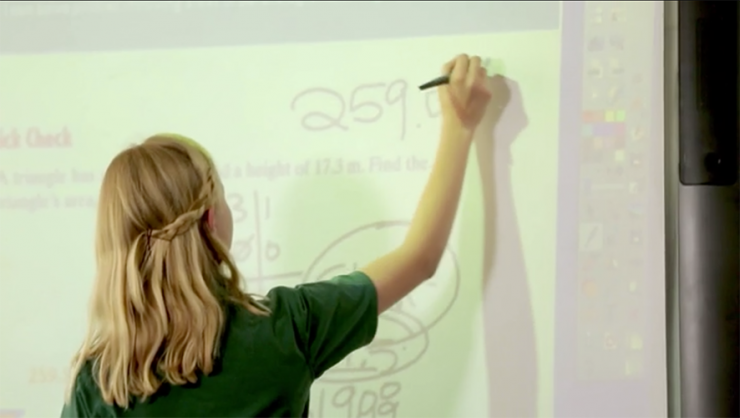# Mathematics## Grade 6 - We build on the skills students have developed in elementary grades.

Practice problems begin to look a lot like algebra. Geometry, probability, statistics, percentages and graphing are covered. Students will also be expected to apply their knowledge of math through complex word problems.

#### Curriculum Highlights

Topics students will explore include the following:

• Decimals, including multiplication and division
• Fractions, including multiplication and division
• Ratios, proportions and percentages
• Algebraic concepts, including variables, patterns, and equations
• Geometry and measurement
• Probability

## Grade 7 -Students expand their understanding and use of algebra.

They will do this by solving equations and inequalities with integers, decimals, fractions, and exponents, as well as explore geometric concepts such as the surface area of prisms and cylinders and the Pythagorean Theorem.

#### Curriculum Highlights

Topics students will explore include the following:

• Algebraic expressions and integers
• Solving one- and two-step equations and inequalities
• Ratios, proportions, and percentages
• Linear functions and graphing
• Area and Volume
• Right Triangles in Algebra

## Grade 8 - We dive deep into algebra.

Increasingly complex functions, equations and inequalities are tackled, along with polynomials and factoring, and quadratic equations.

#### Curriculum Highlights

Topics students will explore include the following:

• Solving and graphing systems of equations and inequalities
• Rational numbers
• Exponents and exponential functions
• Polynomials and factoring